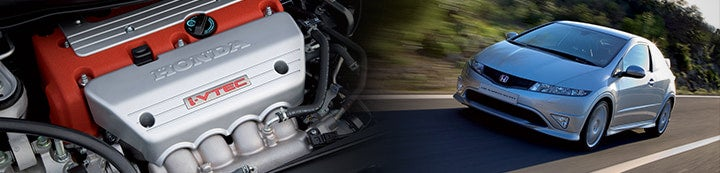1 - 4 of 4 Posts

#### Garet Jax

·
##### Registered
Joined
·
30 Posts
Discussion Starter · ·
How many cc's of displacement does each mm of bore yield?

How many cc's per mm of stroke?

#### Matty

·
##### Registered
Joined
·
245 Posts
The first question would depend on the stroke, the second question would depend on the bore, and both would depend on the number of cylinders in the engine.

Assuming you're talking about a K20A engine, 86mm x 86mm, the answers would be 11.7cc and 5.8cc per cylinder respectively.

The formula to calculate the capacity is:
(Number of cylinders) x pi x (half the bore) x (half the bore) x (stroke)

So for a K20A:
4 x 3.1415926536 (approx) x 4.3 x 4.3 x 8.6 = 1998.2cc

Increasing the bore by 1mm:
4 x 3.1415926536 x 4.35 x 4.35 x 8.6 = 2045cc

Increasing the stroke by 1mm:
4 x 3.1415926536 (approx) x 4.3 x 4.3 x 8.7 = 2021.5cc

Remember all measurements should be in centimetres.

·
Joined
·
3,373 Posts
good info :up:

#### Garet Jax

·
##### Registered
Joined
·
30 Posts
Discussion Starter · ·
Thanks for the formula matty. I meant to include that I was referring to a K20 engine in the first post.

I have one more question: How does each millimeter of bore and stroke translate into power terms?

For instance, since 47 cc's (1mm of cylinder overbore) is approximately a 2% increase in overall displacement, could you assume there would be a 2% increase in torque as well?

1 - 4 of 4 Posts